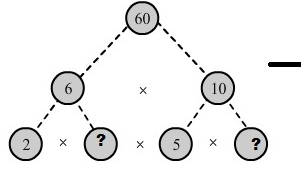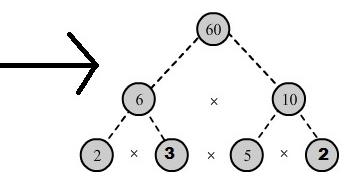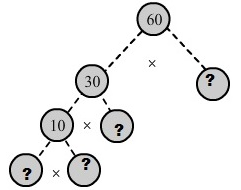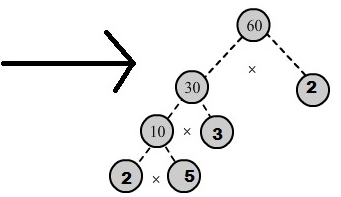Courses

# RD Sharma Solutions -Ex-2.4 Playing With Numbers, Class 6, Maths Class 6 Notes | EduRev

## RD Sharma Solutions for Class 6 Mathematics

Created by: Abhishek Kapoor

## Class 6 : RD Sharma Solutions -Ex-2.4 Playing With Numbers, Class 6, Maths Class 6 Notes | EduRev

The document RD Sharma Solutions -Ex-2.4 Playing With Numbers, Class 6, Maths Class 6 Notes | EduRev is a part of the Class 6 Course RD Sharma Solutions for Class 6 Mathematics.
All you need of Class 6 at this link: Class 6

Q. 1.) In which of the following expressions, prime factorization has been done?

Answer:  (i) 24 = 2 x 3 x 4 is not a prime factorization as 4 is not a prime number.

(ii) 56 = 1 x 7 x 2 x2 x 2 is not a prime factorization as 1 is not a prime number.

(iii) 70 = 2 x 5 x 7 is a prime factorization as 2, 5, and 7 are prime numbers.

(iv) 54 = 2 x 3 x 9 is not a prime factorization as 9 is not a prime number.

Q. 2.) Determine prime factorization of each of the following numbers:

We have:

 2 216 2 108 2 54 3 27 3 9 3 3 1

Therefore, Prime factorization of 216 = 2 x 2 x 2 x3 x 3

(ii) 420

We have:

 2 420 2 210 3 105 5 35 7 7 1

Therefore, Prime factorization of 420= 2 x 2 x 3 x 5 x 7

(iii) 468

We have:

 2 468 2 234 3 117 3 39 13 13 1

Therefore, Prime factorization of 468 = 2 x 2 x 3 x 3 x 13

(iv) 945

We have:

 3 945 3 315 3 105 5 35 7 7 1

Therefore, Prime factorization of 945 = 3 x 3 x 3 x 5 x 7

(v) 7325

We have:

 5 7325 5 1465 293 293 1

Therefore, Prime factorization of 7325= 5 x 5 x 293

(vi) 13915

We have:

 5 13915 11 2783 11 253 23 23 1

Therefore, Prime factorization of 13915 = 5 x 11 x 11 x 23

Q. 3.) Write the smallest 4-digit number and express it as a product of primes.

Answer:  The smallest 4-digit number is 1000.

1000 = 2 x 500

=2 x2 x250

=2 x2 x2 x 125

=2 x2x2x5x 25

=2 x2x2x5x5x5

Therefore, 1000=2 x2 x2x5x5x5

Q. 4.) Write the largest 4-digit number and express it as product of primes.

Answer: The largest 4-digit number is 9999.

We have:

 3 9999 3 3333 11 1111 101 101 1

Hence, the largest 4-digit number 9999 can be expressed in the form of its prime factors as 3 x 3 x 11 x 101.

Q. 5.) Find the prime factors of 1729. Arrange the factors in ascending order, and find the relation between two consecutive prime factors.

Answer: The given number is 1729.

We have:

 7 1729 13 247 19 19

Thus, the number 1729 can be expressed in the form of its prime factors ass 7 x 13 x19.

Relation between its two consecutive prime factors:

The consecutive prime factors of the given number are 7, 13 and 19.

Clearly, 13 – 7 = 6 and 19 – 13 =6

Here, in two consecutive prime factors, the latter is 6 more than the previous one.

Q. 6.) Which factors are not included in the prime factorization of a composite number?

Answer: 1 and the number itself are not included in the prime factorization of a composite number.

Example: 4 is a composite number.

Prime factorization of 4 = 2 x 2.

Q. 7.) Here are two different factor trees for 60. Write the missing numbers:

Answer: (i) Since 6 = 2 x 3 and 10= 5 x 2. We have:(ii) Since 60 = 30 x 2.

30= 10 x 3 and 10 = 5 x 2 we have:103 docs

,

,

,

,

,

,

,

,

,

,

,

,

,

,

,

,

,

,

,

,

,

,

,

,

,

,

,

;